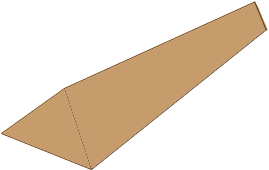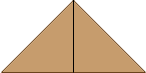SEARCH HOMEMath Central Quandaries & QueriesQuestion from casey: My question is two fold. I want to build a dirt berm that is 95 feet long and 8 feet high which has a 45 degree angle on each side of the slope. My first question is how wide will the berm end up being? Second question is how many cubic yards of dirt will I need to get? Thanks!Ho Casey,

Is your berm to be approximately the shape in my diagram?If so then the fact that the triangular end has base angles of 45o tells me that the height is half the base width.Thus the base width is 16 feet.

The volume is the area of the triangular cross-section times the length of the berm. Since you want the volume in cubic yards I would first convert the measurements to yards. The triangular cross-section has base of width 16/3 yards and height 8/3 yards and hence its area is

(1/2) × base × height = (1/2) × (16/3) × (8/3) = 64/9 square feet.

Hence the volume is (64/9) × (95/3) = 225.2 cubic yards.

HarleyMath Central is supported by the University of Regina and The Pacific Institute for the Mathematical Sciences.